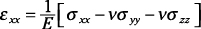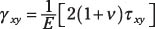##### Mechanics of Materials For DummiesIn mechanics of materials, Hooke's law is the relationship that connects stresses to strains. Although Hooke's original law was developed for uniaxial stresses, you can use a generalized version of Hooke's law to connect stress and strain in three-dimensional objects, as well. Eventually, Hooke's law helps you relate stresses (which are based on loads) to strains (which are based on deformations).

For a three-dimensional state of stress, the normal strain in a given direction (such as x) is a function of the stresses in all three orthogonal directions (usually the Cartesian x-, y-, and z-directions), as shown by this equation:where E is the modulus of elasticity and ν is Poisson's ratio for the material. For a uniaxial stress, two of the stresses in the equation are zero. For a biaxial stress condition, one of the stresses in this equation is zero.

The generalized relationship for Hooke's law for shear in the XY plane can be given as